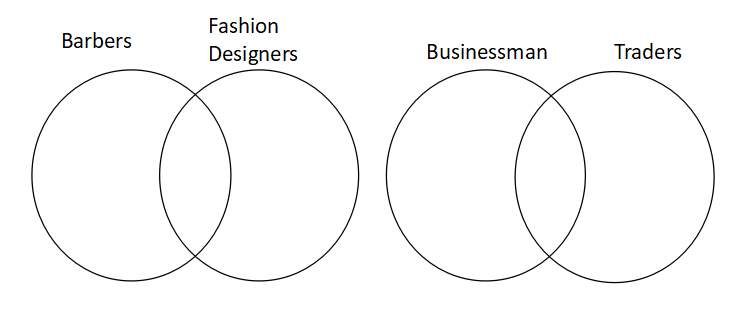### IIFT 2008 Question Paper Question 17

Instructions

Answer the questions based on the following information.

In each question below three statements (I, II, III) are given followed by four conclusions numbered 1, 2, 3 and 4. You have to take the given statements to be true even if they seem to be at variance with commonly known facts. Read all the conclusions and then decide which of the given conclusions logically follows from the given statements, disregarding commonly known facts. Choose the correct options (A & D) presented below.

Question 17

Solution

The given situation is: